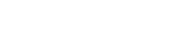# math.sinh

`FLOATmath.sinhFLOATx`

Available inall subroutines.

Computes the hyperbolic sine of its argument `x`.

## Parameters

`x` - Floating point value representing a hyperbolic angle.

## Return Value

Upon successful completion, this function returns the hyperbolic sine of `x`.

If `x` is `math.NAN`, a NaN will be returned.

If `x` is ±0, or `math.POS_INFINITY` or `math.NEG_INFINITY`, `x` will be returned.

If `x` is subnormal, a range error occurs and `x` will be returned.

If the result would cause an overflow, a range error occurs and `math.POS_HUGE_VAL` or `math.NEG_HUGE_VAL` (with the same sign as `x`) will be returned.

### Errors

If the `x` argument is subnormal or if the result would cause an overflow, then `fastly.error` will be set to `ERANGE`.

## Example

``````declare local var.fo FLOAT;
set var.fo = math.sinh(-1);``````

BETA